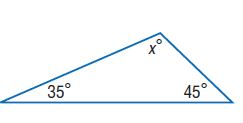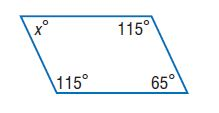Homework Explained - Math Practice 101Dear guest, you are not a registered member. As a guest, you only have read-only access to our books, tests and other practice materials.

As a registered member you can:

Registration is free and doesn't require any type of payment information. Click here to Register.
Go to page:
Chapter 6: Equations and Inequalities; Lesson 1: One-Step Addition And Subtraction Equations

• Question 10

The sum of the measures of the angles of a triangle is 180°. Write and solve an equation to find the missing measure.• Question 11

The sum of the measures of a quadrilateral is 360°. Write and solve an equation to find the missing measure.### H.O.T. Problems Higher Order Thinking

• Question 12

Reason Inductively Write an addition equation and a subtraction equation that have 10 as a solution.

• Type below:
• Question 13

Find the Error Aisha is finding b + 5 = -8. Find her mistake and correct it.• Type below:
• Question 14

Reason Abstractly Suppose x + y = 11 and the value of x increases by 2. If their sum remains the same, what must happen to the value of y?

• Type below:
• Question 15

The Oriental Pearl Tower in China is 1,535 feet tall. It is 280 feet shorter than the Canadian National Tower in Canada. Which equation can be used to find the height of the Canadian National Tower?

• 1,535 + h = 280
• h = 1,535 - 280
• 1,535 = h - 280
• 280 - h = 1,535

Yes, email page to my online tutor. (if you didn't add a tutor yet, you can add one here)